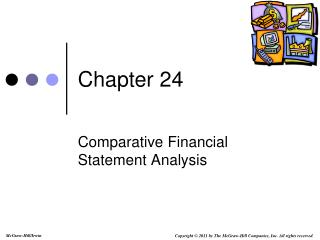# Chapter 24 - PowerPoint PPT PresentationDownload PresentationChapter 24

Chapter 24Download Presentation## Chapter 24

- - - - - - - - - - - - - - - - - - - - - - - - - - - E N D - - - - - - - - - - - - - - - - - - - - - - - - - - -
##### Presentation Transcript

1. Chapter 24 Comparative Financial Statement Analysis

2. LO1 Learning Objective 1Explain the purpose of analysis. Involves transforming data Reduces uncertainty Financial statement analysis helps users make better decisions. Application of analytical tools

3. LO2 Learning Objective 2Identify the building blocks of analysis. Liquidity and efficiency-ability to meet short-term obligations and to efficiently generate revenues. Solvency-ability to generate future revenues and meet long-term obligations. Profitability-ability to provide financial rewards sufficient to attract and retain financing. Market prospects-ability to generate positive market expectations. Basic information for our analysis comes from the financial statements and the notes to those statements. In some cases, we may need to develop supplemental information to complete our analysis.

4. LO3 Learning Objective 3Describe standards for comparisons in analysis. When we complete our analysis, it is essential to compare the results we obtained to previous years within our company, those of our competitors, other companies in our same industry, and general financial market guidelines. • Intracompany • Competitor • Industry • Guidelines

5. LO4 Learning Objective 4Identify the tools of analysis. Horizontal Analysis Comparing a company’s financial condition and performance across time. Vertical Analysis Comparing a company’s financial condition and performance to a base amount. Ratio Analysis Measurement of key relations between financial statement items.

6. Calculate Change in Dollar Amount Learning Objective 5 Explain and apply methods of horizontal analysis. LO5 Since we are measuring the amount of the change between 2009 and 2010, the dollar amounts for 2009 become the “base” period amounts. The first task that we face is establishing the base year and then calculating changes in reference to the base. In horizontal analysis, we use the oldest year shown as the base year and determine the dollar change between the base year and the current year. For this example, 2009 is its base year. Dollar change Analysis period amount Base period amount = –

7. Calculate Change as a Percent LO5 Comparative Statements Once we establish the base year, we can calculate the percentage change by dividing the dollar change by the base year amount and multiplying by 100. Percent change Analysis period amount Base period amount × 100 = \$12,000 – \$23,500 = \$(11,500) (\$11,500 ÷ \$23,500) × 100 = 48.9% For the dollar change in cash, we subtract the base year amount of \$23,500 from the current year amount of \$12,000 to determine the change in cash was a negative \$11,500. We know the percentage change will be negative. To determine the percentage change, we divide the dollar change of \$11,500 by the base year balance of \$23,500, and multiply by 100%. The percentage change is a decrease in cash of 48.9%.

8. Calculate Common-size Percent LO6 Financial Statement Base Amount Balance Sheet Total Assets Income Statement Revenues Learning Objective 6Describe and apply methods of vertical analysis. Common-size percent Analysis amount Base amount × = 100 Common size financial statements are prepared for a single period. We express all items on the statement in terms of one component of that statement. For the income statement, we normally express all items as a percent of revenues. For the balance sheet, we generally express all items as a percent of total assets.

9. LO6 We want to express all line-items on the financial statement in terms of total assets, so we set total assets equal to 100%. We calculate the percentage of total assets made up of cash and cash equivalents. We divide the total cash and cash equivalents for 2009 by the total assets for 2009, and multiply the result by 100%. At the end of 2009, cash and cash equivalents make up 3.8% of total assets. The same measure shows the percentage for 2008 to be 8.1%. (\$23,500 ÷ \$289,700) × 100 = 8.1% (\$12,000 ÷ \$315,000) × 100 = 3.8% You can see that accounts receivable make up 19% of total assets in 2010, and 13.8% in 2009. There was a drop in total current assets and an increase in property and equipment from 2009 to 2010.

10. LO6 Here is our vertical analysis for the liabilities and equity side of the balance sheet.

11. LO7 Learning Objective 7Define and apply ratio analysis. Ratio Analysis Ratios are among the more widely used tools of financial analysis because they help us uncover conditions and trends. A ratio expresses a mathematical relation between two qualities. It can be expressed as a percent, rate, or proportion. For example the current ratio: Current assets Current liabilities For more ratios see Exhibit 24.7 in your book. Current ratio ═

12. LO8 Learning Objective 8Summarize and report results of financial statement A good analysis report usually consists of six sections: Executive summary-brief focus on important analysis results and conclusions. Analysis overview-background on the company, its industry, and its economic setting. Evidential matter-financial statements and information used in the analysis, including ratios, trends, comparisons, statistics, and all analytical measures assembled. Assumptions-identification of important assumptions regarding a company’s industry and economic environment, and other important assumptions for estimates. Key factors-list of important favorable and unfavorable factors, both quantitative and qualitative. Inferences-forecasts, estimates, interpretations, and conclusions drawing on all sections of the report.

13. End of Chapter 24